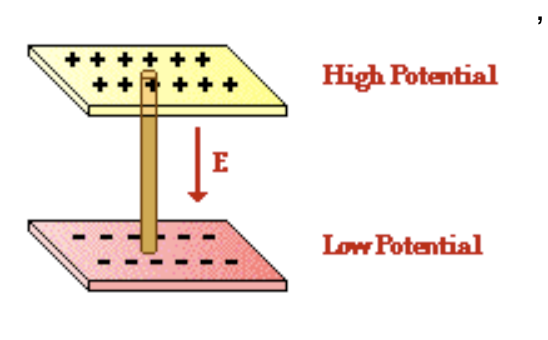A set of questions that came up while studying DC Circuits.

At least temporary physics questions.

Where does the charge come from? Is it just an inherent property of the material?Two battery setup? The positive terminal of battery 1 is attached to something and the negative terminal of battery 2 receives?

Can we uncharge a material?

Moving charges generate a magnetic field and variations in a magnetic field produce an electric current. Do moving masses, or gravitational fields produce anything? Is it too coupled with magnetism and electricity.

General questions:

Why do these equations work: P=IV and I=V/R?
What are the possible sources of EM radiation?
What is the relationship between the direction of the movement of charge and the EM field produced from their movement? In terms of directionality and strength.
How does the center of balance of a system move when the masses in the system rearrange?
Why is/how is the force felt by something being rotated dependent on the length of the arm used to rotate it?
How do fundamentals add and subtract?
Why does light refract when entering substances of a different densities? Is a wave or particle perspective needed to understand this?

More generally/ simply:

Why/how does light bend when going through a lens/mirror?
Why does the angle of incidence=angle of reflection? Both optically and physically?